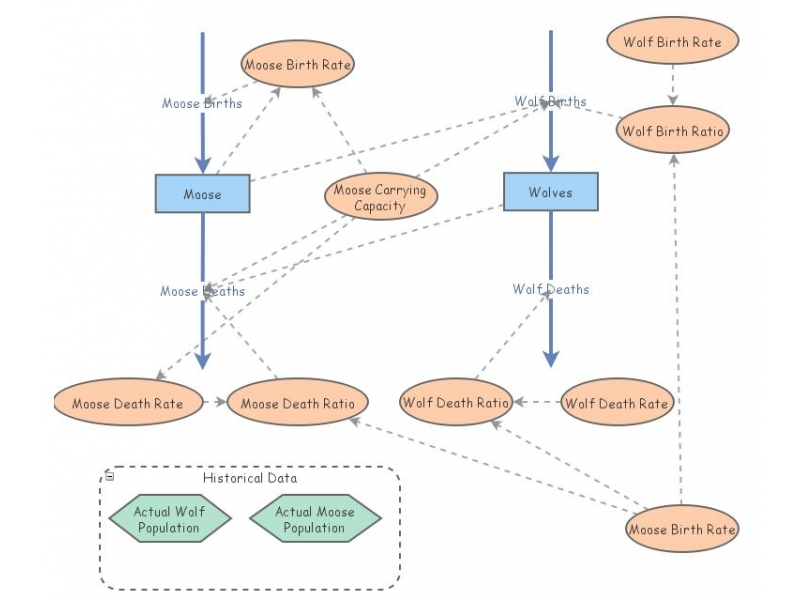Sleek, non-dimensionalized Isle Royale: Predator/Prey Model for Moose and WolvesThis non-dimensionalized, sleekest most neatest model illustrates predator prey interactions using logistic growth for the moose population, for the wolf and moose populations on Isle Royale.

Thanks Scott Fortmann-Roe for the original model.

I've added in an adjustment to handle population sizes, by dividing by moose carrying capacity.

Time is scaled by the moose birth parameter:
tau=bm*t

There are therefore only three parameters left to account for any dynamics:

beta = bw/bm (relative wolf to moose births)
delta = dm/bm (relative death to birth ratio for moose)
gamma = dw/bm (wolf deaths to moose births)

The equations are thus

dM/dtau = M [ (1-M) - delta W ]
dW/dtau = W [beta M - gamma ]

There is a stable equilibrium pair of population values, relative to the carrying capacity:

M^* = gamma / beta
W^* = (1-gamma / beta) / delta

I have a sleek version with a logistical growth term for the moose, at

http://www.nku.edu/~longa/classes/2018spring/mat375/mathematica/Moose-n-Wolf-InsightMaker-sleek.nb